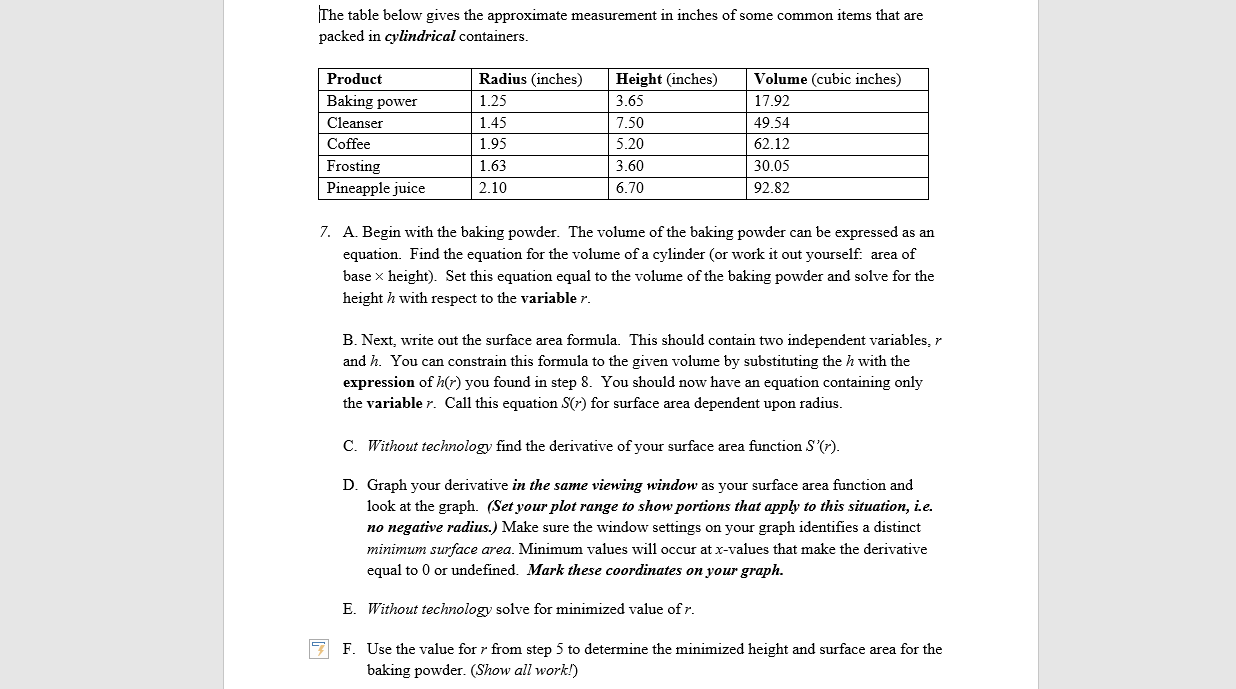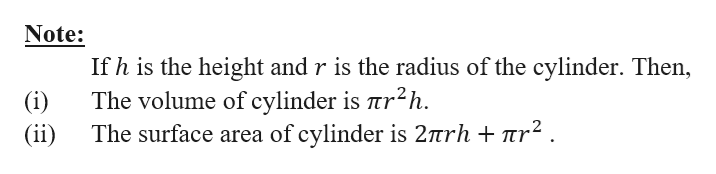# The table below gives the approximate measurement in inches of some common items that arepacked in cylindrical containers.Radius (inches)Volume (cubic inches)ProductHeight (inches)Baking power1.253.6517.921.457.50Cleanser49.54Coffee1.955.2062.12Frosting1.633.6030.052.106.70Pineapple juice92.827. A. Begin with the baking powder. The volume of the baking powder can be expressed as anequationFind the equation for the volume of a cylinder (or work it out yourself. area ofbase x height). Set this equation equal to the volume of the baking powder and solve for theheight h with respect to the variable r.B. Next, write out the surface area formula. This should contain two independent variables, rand h. You can constrain this formula to the given volume by substituting the h with theexpression of h(r) you found in step 8. You should now have an equation containing onlythe variable r. Call this equation S(r) for surface area dependent upon radius.Without technology find the derivative of your surface area function S'(7).C.D. Graph your derivative in the same viewing window as your surface area function andlook at the graph. (Set yourplot range to show portions that apply to this situation, i.e.no negative radius.) Make sure the window settings on your graph identifies a distinctminimum surface area. Minimum values will occur at x-values that make the derivativeequal to 0 or undefined. Mark these coordinates on your graph.EWithout technology solve for minimized value ofrF. Use the value for r from step 5 to determine the minimized height and surface area for thebaking powder. (Show all work!)

Question
14 viewshelp_outlineImage TranscriptioncloseThe table below gives the approximate measurement in inches of some common items that are packed in cylindrical containers. Radius (inches) Volume (cubic inches) Product Height (inches) Baking power 1.25 3.65 17.92 1.45 7.50 Cleanser 49.54 Coffee 1.95 5.20 62.12 Frosting 1.63 3.60 30.05 2.10 6.70 Pineapple juice 92.82 7. A. Begin with the baking powder. The volume of the baking powder can be expressed as an equation Find the equation for the volume of a cylinder (or work it out yourself. area of base x height). Set this equation equal to the volume of the baking powder and solve for the height h with respect to the variable r. B. Next, write out the surface area formula. This should contain two independent variables, r and h. You can constrain this formula to the given volume by substituting the h with the expression of h(r) you found in step 8. You should now have an equation containing only the variable r. Call this equation S(r) for surface area dependent upon radius. Without technology find the derivative of your surface area function S'(7). C. D. Graph your derivative in the same viewing window as your surface area function and look at the graph. (Set yourplot range to show portions that apply to this situation, i.e. no negative radius.) Make sure the window settings on your graph identifies a distinct minimum surface area. Minimum values will occur at x-values that make the derivative equal to 0 or undefined. Mark these coordinates on your graph. E Without technology solve for minimized value ofr F. Use the value for r from step 5 to determine the minimized height and surface area for the baking powder. (Show all work!) fullscreen
check_circle

Step 1

Hi there! The question is having many sub-parts. Since no specification is given, only first three sub-parts are being answered. If you want the other parts to be answered, then kindly re-submit the question with specification.

Step 2

The given table is containing the information about product and the approximate measurement in inches that are packed in cylindrical containers.help_outlineImage TranscriptioncloseNote: If h is the height and r is the radius of the cylinder. Then, The volume of cylinder is tr2h. (i) The surface area of cylinder is 2nrh + 7nr2 . (ii) fullscreen
Step 3

A. Beginning with the Baking powder.

Given that the volume of ...

### Want to see the full answer?

See Solution

#### Want to see this answer and more?

Solutions are written by subject experts who are available 24/7. Questions are typically answered within 1 hour.*

See Solution
*Response times may vary by subject and question.
Tagged in

### Derivative